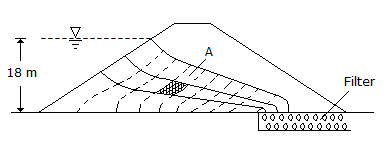# Civil Engineering - UPSC Civil Service Exam Questions

26.

In the schematic flownet shown in the given figure the hydraulic potential at point A isA. 5 m of water B. 12 m of water C. 15 m of water D. 25 m of water

Explanation:

No answer description available for this question. Let us discuss.

27.

A 4-hour rainfall in a catchment of 250 km2 produces rainfall depths of 6.2 cm and 5.0 cm in successive 2-hour unit periods. Assuming the φ index of the soil to be 1.2 cm/hour, the run-off volume in ha-m will be

 A. 16 B. 22 C. 1600 D. 2200

Explanation:

No answer description available for this question. Let us discuss.

28.

Consider the following statements :
The critical path in a network plan of a project
1. helps in planning efficient time schedule
2. indicates the shortest path in time
3. helps in crashing the project judiciously
4. helps in encouraging discipline in execution.
Of these statements :

 A. 1, 3 and 4 are correct B. 1, 2, 3 and 4 are correct C. 1 and 4 are correct D. 2 and 3 are correct

Explanation:

No answer description available for this question. Let us discuss.

29.

Which of the following pairs are correctly matched ?
1. Alpha iron....non magnetic
2. Beta iron....strongly magnetic
3. Gamma iron....non-magnetic and capable of dissolving carbon.
4. Delta iron....non-magnetic and absorbs very little carbon.
Select the correct answer using the codes given below :

 A. 3 and 4 B. 1 and 2 C. 1, 3 and 4 D. 2 and 4

Explanation:

No answer description available for this question. Let us discuss.

30.

The height of a hydraulic jump in a stilling pool was found to be 10 cm in a model with lp/lm = 36. The prototype jump height would be

 A. 0.6 m B. 3.6 m C. 21.6m D. indeterminable for want of adequate data